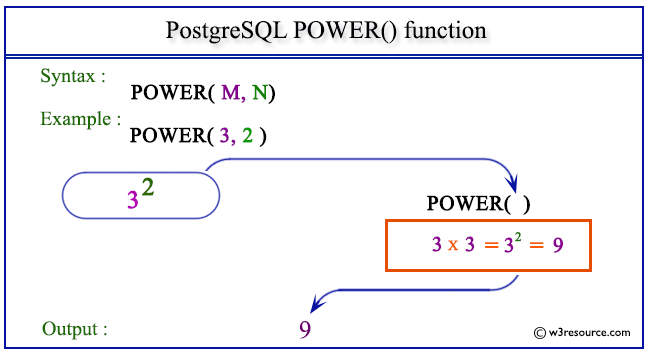# PostgreSQL POWER() function

## POWER() function

The PostgreSQL power() function is used to return the value of one number raised to the power of another number, provided in the argument.

Syntax:

```power()
```

PostgreSQL Version: 9.3

Pictorial presentation of PostgreSQL POWER() functionExample 1: PostgreSQL POWER() function :

Code:

``````SELECT POWER(7.0,3) AS "7 raised to the power of 3";
```
```

Sample Output:

``` 7 raised to the power of 3
----------------------------
343.0000000000000000
(1 row)
```

Example 2: PostgreSQL POWER() function

Code:

``````SELECT POWER(7,3) AS "7 raised to the power of 3";
```
```

Sample Output:

``` 7 raised to the power of 3
----------------------------
343
(1 row)
```

Previous: PI function